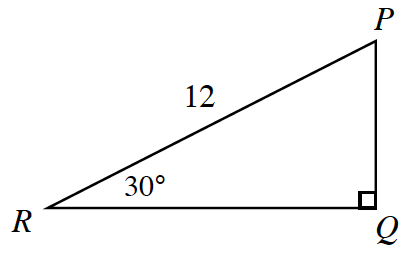### Home > PC3 > Chapter 1 > Lesson 1.2.1 > Problem1-73

1-73.Use the triangle at right to answer the following questions.

1. What kind of a special triangle is this?

What are the measures of the three angles?

2. What is the exact length of $PQ$?

Set up a ratio with the $\sin 30º$.

3. What is the exact length of side $QR$?

$6\sqrt{3}$

4. Write and simplify the sine ratio for $∠P$. Leave in simplified radical form.

$\sin(P)=\frac{QR}{PR}$